# Introduction and Use Case:

Effective Per GB Price is a crucial part of any cost optimization exercise against your environment. How do you find your Effective Per GB Price and how do you use it to calculate how much stuff costs?

# In this post we will:

• Identify deployment region for both Sentinel and Log Analytics Workspace (LAW)
• Identify the Sentinel and Log Analytics Workspace Commitment Tiers
• Identify and Calculate the Sentinel and LAW Effective per GB rates
• What if Sentinel and LAW are on Different Commitment Tiers?
• Plug Rates into KQL Queries to Calculate Costs

# Identify deployment region for both Sentinel and Log Analytics Workspace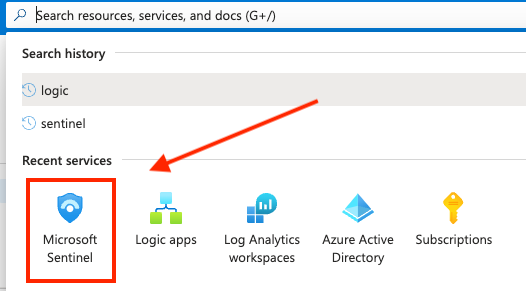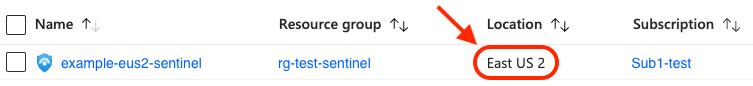Take note of the location, we’ll need it later

# Identify Sentinel and Log Analytics Workspace Commitment Tiers

To determine your Sentinel commitment tier, search “Sentinel” in the top navbar then select your workspace and click on the Settings blade, illustrated in the following screenshots: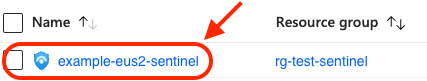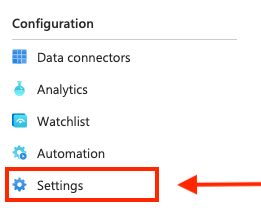Pro Tip: Shortcut to LAW from Settings blade in Sentinel: To skip searching for LAW in the navbar and several subsequent clicks to get to the same place. While in Sentinel, you can quickly swith to LAW using this shortcut in the Settings blade: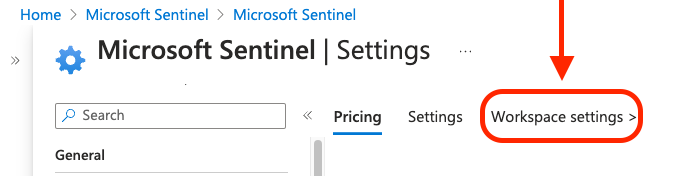Then you can go directly to the Usage and estimated costs blade: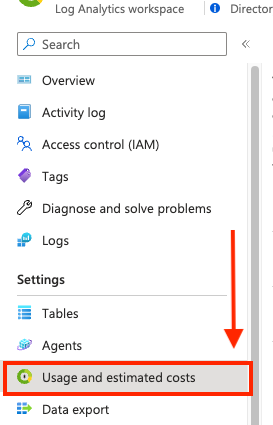# Calculate the Sentinel and Log Analytics Workspace Effective per GB Rates

In a browser, navigate to Microsoft Sentinel Pricing and select the matching region from the dropdown: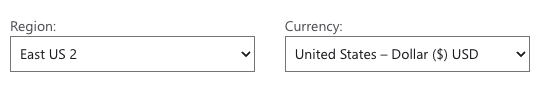If both Sentinel and Log Analytics Workspace are set to the same commitment tiers (both are Pay-as-you-go or 100GB for example), then this next part is easy. If you were on Pay-as-you-go, you could determine Sentinel and Log Analytics Workspace costs separately using their respective per GB rates, or you could calculate your overal ingest cost using the combined effective rate per GB value for your region.

If both tiers are set to 100GB per Day, then divide the cost per day by the number of GBs for that tier. For example:

Sentinel:

• ($100/day) % (100GB/day) = ($100 % 100GB) = $1.00 / GB Log Analytics Workspace: • ($196/day) % (100GB/day) = ($196 % 100GB) =$1.96 / GB

To prove these rates are accurate, we can independently calculate the total effective per GB rate and verify it against the offical Microsoft Sentinel Pricing page, illustrated below:

Effective per GB rate:

• (Sentinel rate + LAW rate) = ($1.00 +$1.96) = $2.96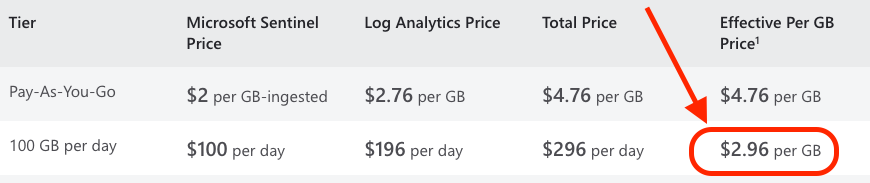# What if Commitment Tiers Don’t Match/My Price isn’t Listed? This isn’t typical, but it happens. Suppose your Sentinel Commitment Tier is Pay-as-you-go and your Log Analytics Workspace is on the 100GB / day commitment tier. Obviously the Sentinel per GB cost is$2 because they’re on the Pay-as-you-go plan. The Log Analytics Workspace rate is per day and needs to be converted to per GB. Just like we did in the previous example, we divide the cost per day by the number of GBs for the Log Analytics price:

Log Analytics Workspace:

• ($196/day % 100GB/day) = ($196 % 100GB) = $1.96 / GB All we have to do next is combine this with the Sentinel cost per GB for a total Effective Per GB Price: • Sentinel ($2.00/GB) + LAW ($1.96) = Effective Per GB Price ($3.96)

In this example where Sentinel is set to Pay-as-you-go and LAW is set to 100GB / day, the effective per GB rate is \$3.96

# Plug Rates into KQL Queries to Calculate Costs

You can now plug in the above rates to confidently calculate either the Sentinel or LAW cost of say, a table or an EventID etc. Here’s an example query breakdown that uses the custom rate we calculated earlier to calculate the ingest cost of the SecurityEvent table (you can swap out SecurityEvent and apply this to other tables to see what else you’re spending your money on):

let rate = 3.96;                            //<-- Effective Cost per GB
SecurityEvent		             		        //<-- Query the SecurityEvent table
| where TimeGenerated > ago(1h)		         //<-- Query the last hour
| where EventID == 8002			                //<-- Query for EventID 8002
| summarize GB=sum(_BilledSize)/1000/1000/1000	//<-- Summarize billable volume in GB using the _BilledSize table column
| extend cost = GB*rate                   //<-- Multiply total GBs for the month by the effective rate (defined in first line of query)


Check out my KQL Repo for more KQL queries where you can plug in the rate to calculate cost, and more!

# Summary:

In this post, we

• Identified our deployment region for both Sentinel and Log Analytics Workspace
• Identified our Sentinel and Log Analytics Workspace Commitment Tiers
• Calculated the Sentinel and LAW Effective per GB rates
• What if Sentinel and LAW are on Different Commitment Tiers?
• Plugged Rates into KQL Queries to Calculate Costs